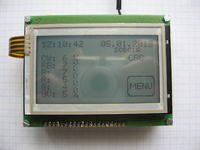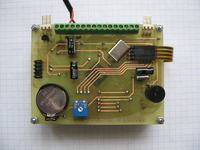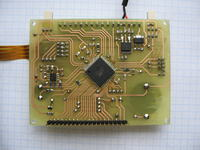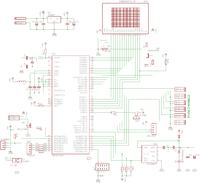# LED touch controller – lamp driver

Status
Not open for further replies.

#### VermesThis LED lamp driver was based on Atmega128 and it uses graphical display with the touch pad. Program was written in Bascom and occupies 45%, the half of which consists of graphics and animations.

There are six independently controlled hardware PWM channels. It is possible to set the time of sunrise and sunset as well as enabling and disabling times of the individual channels. You can also add some extras such as weather effects (cloudiness, storms, moon phases).

The controller controls current pulse drivers MBI6651, but it can work with every drivers that have the input for PWM signal.

The schematic may slightly differ from the PCB, because some improvements were introduced, the PCB is double-sided.

Code:
Code:
'@@@@@@ odczyt touchpada @@@@@@
If F100ms = 1 Then
Config Pinf.4 = Output
Config Pinf.6 = Output
Reset Portf.4
Set Portf.6
Ddrf.5 = 0
Ddrf.7 = 0
Waitms 10
Y = 1024 - Y
*
Config Pinf.5 = Output
Config Pinf.7 = Output
Set Portf.5
Reset Portf.7
Ddrf.4 = 0
Ddrf.6 = 0
Waitms 10
X = 1024 - X
*
Select Case X
Case 180 To 350 : Kolumna = 10
Case 351 To 510 : Kolumna = 20
Case 511 To 690 : Kolumna = 30
Case 691 To 840 : Kolumna = 40
Case Else Kolumna = 0
End Select
*
Select Case Y
Case 280 To 390 : Rzad = 1
Case 391 To 525 : Rzad = 2
Case 526 To 650 : Rzad = 3
End Select
*
If Klucz > 10 Then
Keyarray(keylus) = Klucz
Incr Keylus
If Keylus > 3 Then Keylus = 1
If Keyarray(1) = Keyarray(2) Then
If Keyarray(2) = Keyarray(3) Then
Sound Buzer , 1 , 65000
Set Buzer
Przycisk = Klucz
Autooff = Wygaszacz_czas
Wygaszacz_ekran = 0
Animacja = 0
If Autooff = Wygaszacz_czas Then
If Podswietlenie_blok = 0 Then
Select Case Podswietlenie_aktywne
Case Is = 0 : Ocr0 = 0
Case Is = 1 : Ocr0 = 25
Case Is = 2 : Ocr0 = 50
Case Is = 3 : Ocr0 = 75
Case Is = 4 : Ocr0 = 100
Case Is = 5 : Ocr0 = 125
Case Is = 6 : Ocr0 = 150
Case Is = 7 : Ocr0 = 175
Case Is = 8 : Ocr0 = 200
Case Is = 9 : Ocr0 = 250
End Select
End If
End If
Reset F100ms
End If
End If
End If
End If

Pictures:Video:

Link to original thread (useful attachment) - LED Kontroler Touch - sterownik lampy

Status
Not open for further replies.